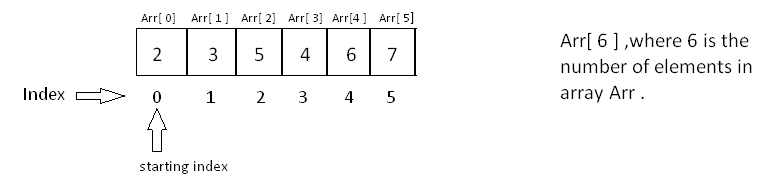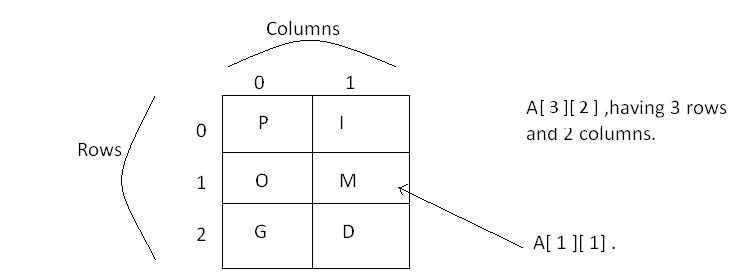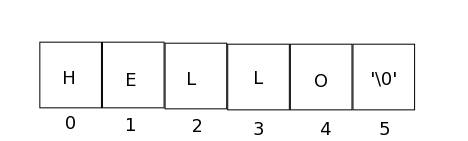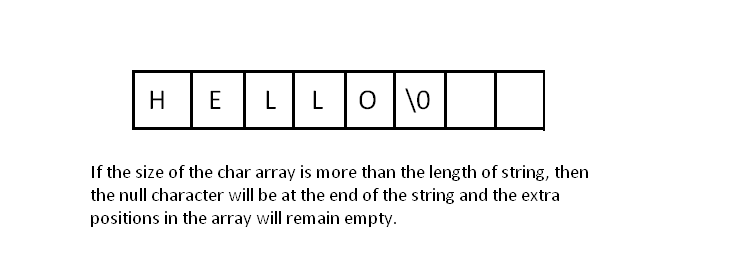500
Arrays and Strings - Code Monk
Arrays
Strings
CodeMonk

An array is a sequential collection of variables of same data type which can be accessed using an integer as index, that generally starts from 0. It stores data elements in a continuous memory location. Each element can be individually referenced with its respective index.

1-Dimensional array: It is a linear array that stores elements in a sequential order. Let us try to demonstrate this with an example: Let us say we have to store integers 2, 3, 5, 4, 6, 7. We can store it in an array of integer data type. The way to do it is:

 dataType nameOfTheArray [sizeOfTheArray];
int Arr;


Here Arr is the name of array and 6 is the size. It is necessary to define the size array at compile time.

To store the above elements in the array:

dataType nameOfTheArray [ ] = {elements of array };

int Arr [ ] = { 2, 3, 5, 4, 6, 7 };


Since, the indexing of an array starts from 0, if the 4th element needs to be accessed, Arr [ 3 ] will give you the value of the 4th element. Similarly, nth element can be accessed by Arr[n-1].Multidimensional array: It can be considered as an array of arrays. The most commonly used multidimensional array is 2-dimensional array. It stores the elements using 2 indices, which give the information, in which row and which column a particular element is stored. A 2D array is essentially a matrix.

Declaration:

char A[ 3 ] [ 2 ] ;


Here, A is a 2D array of character data type. The array contains 3 rows and 2 columns.

This array can be used to store 3 words of length 2, for example - PI, OM, GD. These words will be stored in the array as shown in the figure:Here rows and columns start with 0 index. If you have to access ‘M’ character, you can access it by A[ 1 ] [ 1 ].

Let’s experiment with the code below:

#include<stdio.h>
#include<iostream>

using namespace std;
int main( ) {
int Arr; // declaring Arr
Arr[ ] = { 2, 3, 5, 4, 6, 7 }; // Initializing Arr by storing some elements in it.
char A [ 3 ] [ 2 ]; // declaring a 2D array
A [ 3 ] [ 2 ] = { { ‘P’, ‘I’} , {‘O’, ‘M’ } , {‘G’, ‘D’} } ;

// Accessing and printing the 3rd element of the first array.
printf(“The third element of Arr is\n ”,  Arr[ 2 ]);

// Printing all the elements of Arr
for (int i = 0; i < 6 ; i ++ )
printf(“%d “, Arr[i]);
printf(“\n”);

// Printing all the element of A[ ][ ]
printf(“All the elements of 2D array A[ ][ ] are : \n”);

for( int i = 0 ; i < 3; i++ ) {
for(int j= 0 ; j < 2 ; j++ ) {
printf(“%c “, A[i][j]);
}
printf(“\n”);
}
return 0;
}


Output :

The third element of Arr is 5
2 3 5 4 6 7
All the elements of 2D array A[ ][ ] are :
P I
O M
G D

Time for a surprise quiz on array? Great. Here it is. Let’s say that you have an array: A[ 6 ] = {2, 5, 6, 4, 7, 9} and you have to find the sum of elements between index 2 to 4. How will you do that ?

You can simply run a loop from starting index to ending index and can store the sum in the particular variable. Let’s implement this in C++:

#include<iostream>
#include<stdio.h>
using namespace std;
int main ( ) {
int A[ 6 ] = {2, 5, 6, 4, 7, 9 } ;

// Take a variable of integer type to store sum and initialize it to 0 .
int SUM = 0;

/* Run a loop from starting index to ending index. */

for( int i = 2 ; i <= 4 ;i++ ) {
SUM = SUM + A[ i ] ;
}
printf(“The required output is:  %d\n”, SUM);
return 0;
}


Output :
The required output is: 17

A string is essentially a sequence of characters. In other words, we can say that string is an array of character data type. An instance of a string is called a string literal . They are enclosed in double quotes (“”) . For example “HackerEarth” is a called a string literal. There are two types of string in C++: C-style string String class type

C-style strings are one dimensional array of characters. However they have a special property, they end with a null character (‘\0’) to signify the end of the string.

Declaration:

char str[ 6 ];


Initialization: A character array can be initialized like any other normal array

char str[ 6 ] = { ‘H’, ‘E’, ‘L’, ‘L’, ‘O’ };


or it can directly be initialized using a string literal

char str[ ] = “HELLO”;str is a string which has an instance (string literal) “HELLO”. In spite of only 5 characters, the size of the string is 6, because the null character represents the end of the string. The null character is automatically added by the compiler as long as the size of the array is at least one larger than the size of the string. It is not mandatory that the array size (N) be exactly greater than the size of the string (n) by 1 i.e. N >= n + 1To take C-style string as an input you can simple use scanf() with ‘%s’ format specifier or we can also use cin. It will take input until we press a space or enter. For example:

char a;
scanf(“%s”, a);


Similarly you can print the C-style string with the printf() with ’%s’ format specifier or cout.

printf(“%s\n”, a);


Now let us talk about C++ string data type. It is different than your usual C-style string function in many ways. In C, strings are just character arrays which end with a null character. However in C++ strings, one need not bother about the dimensions of the array, or the null character. C++ provides an internal class type which is used for working with strings. Strings can be declared and initialized as follows

Declaration:

string str;


Initialization:

 string str = “HELLO”;


Please note that even though C++ strings are a separate class type, their characters can still be referenced using a 0 based indexing, just like C strings. For example in the above example str[ 2 ] is the character ‘L’ and one can iterate over the string just like a normal array.

Let us now consider some functions which we can perform on strings. In C there are some predefined functions that can be used to do common operations on strings like copy, concatenation, comparison and finding the length. An important thing to note is that all the functions which are used in C-style string function can also be applied on C++ string.

Copy: Copy one string to other. C-style string function:

 strcpy(s1, s2);
C++ string:

s2 = s1;


Length: Finding the length of the string. C-style string function: strlen(s1); C++ string:

s1.size();


Concatenate: Adding one string at the end of the other. C-style string function: strcat(s1, s2);

C++ string:

s1 += s2;


Compare: Comparing two string if they are equal or not. C-style string function:

strcmp(s1, s2);


C++ string:

s1 == s2


NOTE: All the above C-style string functions are in header file of C++.

To take string data type as an input you cannot use scanf(). We need to use cin. It will take input until we press a space or enter. For example:

string a;
cin >> a;


Similarly you cannot print the string data type with the printf() directly. Either we can use cout or we can convert the string data type into the C-style string using c_str() function and then use printf().

string a = “HELLO”;
cout << a << endl;
printf(“%s\n”, a.c_str());


Consider the problem of finding the length of a string without using any inbuilt functions.

To compute the length of the string, we should traverse from the beginning till when the null character is encountered. Since the null character represent the end of the string - thus, helping us know that we have reached the end of the string.

#include <stdio.h>
#include <iostream>
#include <cstring>

using namespace std;

int main() {
char str[] = “HELLO”;                   // Declaration and Initialization
int length1 = 0, length2;

// Loop from beginning till we reach null character.
for(int i = 0 ; str[i] != ‘\0’; ++i)
length1++;

length2 = strlen(str);
printf(“The length of the string str is: %d\n”, length1);
printf(“The length of the string str is: %d\n”, length2);
return 0;
}


Output:
The length of the string str is: 5
The length of the string str is: 5

str has the instance “HELLO”. We start from the beginning i.e., s[ 0 ] which is equal to ‘H’ and compare it with null character. Then, we will continue this by comparing each character in the string with the null character.

Now, let’s give you some problems to ponder on before the contest next week:

Q: How will you copy one string to another without using any inbuilt libraries?
Q: How will you insert an element into an array at a given particular position?
Q: How will you find out the number of occurrences of a particular character in a string?